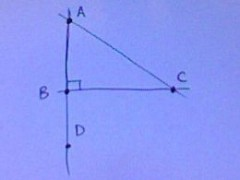Triangle ABC Has A Right Angle Which Is Angle ABC And A Line Ifs Formed Called Line Segment ABD.if Line Segment AC Is Perpendicular To Line Segment DB And The Measurement Of Angle CBD=125 Degrees,then The Measurement Of Angle A Equals What?